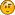# Mathematical formulae

• 9 Replies
• 1690 Views##### Mathematical formulae
« on: September 29, 2016, 10:48:36 AM »
Is there a method of inserting mathematics in a post?

?

#### origamiscienceguy

• 2138##### Re: Mathematical formulae
« Reply #1 on: September 29, 2016, 10:58:20 AM »
Toucan just type it.

There are subscript and superscript tools, so you can try your best. Or you could post an image of your equations.
I wonder how obnoxious I can make my signature?#### Space Cowgirl

• MOM
• 49249
• Official FE Recruiter##### Re: Mathematical formulae
« Reply #2 on: September 29, 2016, 11:00:20 AM »
According to John Davis you can use  jsTex bbcode. I don't know how that works!

Oh, here I found the post.

[jsTex]d(P_1,P_2) = \cos^{-1} \left( \sin \left(\tan^{-1} \left(\frac{x_1^2 + y_1^2 -1}{2x_1} \right) \right) \sin \left(\tan^{-1} \left(\frac{x_2^2 + y_2^2 -1}{2x_2} \right) \right) +\cos \left(\tan^{-1} \left(\frac{x_1^2 + y_1^2 -1}{2x_1} \right) \right) \cos \left(\tan^{-1} \left(\frac{x_2^2 + y_2^2 -1}{2x_2} \right) \right) \cos \left|\tan^{-1} \left( \frac{y_1}{x_1}  \right) - \tan^{-1} \left( \frac{y_2}{x_2} \right) \right| \right)[/jsTex]

Use the jsTex bbcode!

That looks super fricking complicated.
I'm sorry. Am I to understand that when you have a boner you like to imagine punching the shit out of Tom Bishop? That's disgusting.##### Re: Mathematical formulae
« Reply #3 on: September 30, 2016, 09:54:31 PM »
Thank you, I'll have a pop at that.##### Re: Mathematical formulae
« Reply #4 on: October 04, 2016, 01:19:45 AM »
According to John Davis you can use  jsTex bbcode. I don't know how that works!

Oh, here I found the post.

[jsTex]d(P_1,P_2) = \cos^{-1} \left( \sin \left(\tan^{-1} \left(\frac{x_1^2 + y_1^2 -1}{2x_1} \right) \right) \sin \left(\tan^{-1} \left(\frac{x_2^2 + y_2^2 -1}{2x_2} \right) \right) +\cos \left(\tan^{-1} \left(\frac{x_1^2 + y_1^2 -1}{2x_1} \right) \right) \cos \left(\tan^{-1} \left(\frac{x_2^2 + y_2^2 -1}{2x_2} \right) \right) \cos \left|\tan^{-1} \left( \frac{y_1}{x_1}  \right) - \tan^{-1} \left( \frac{y_2}{x_2} \right) \right| \right)[/jsTex]

Use the jsTex bbcode!

That looks super fricking complicated.

Jane's a boss, I hope the angry globuralists didn't chase her off, I enjoyed her posts.
Quote from: Stash
I'm anti-judaism.

Quote from: Space Cowgirl
Whose narrative is it to not believe the government?

Quote from: Wolvaccine
speech should be a privilege. Not a right.#### markjo

• Content Nazi
• The Elder Ones
• 42526##### Re: Mathematical formulae
« Reply #5 on: October 06, 2016, 11:34:52 AM »
Since when do FE'ers use mathematical formulas?Science is what happens when preconception meets verification.
Quote from: Robosteve
Besides, perhaps FET is a conspiracy too.
Quote from: bullhorn
It is just the way it is, you understanding it doesn't concern me.#### hoppy

• Flat Earth Believer
• 11803##### Re: Mathematical formulae
« Reply #6 on: October 07, 2016, 08:27:25 AM »
Since when do FE'ers use mathematical formulas?Since the beginning of time, thank you very much.#### markjo

• Content Nazi
• The Elder Ones
• 42526##### Re: Mathematical formulae
« Reply #7 on: October 07, 2016, 10:18:49 AM »
Since when do FE'ers use mathematical formulas?Since the beginning of time, thank you very much.
Do you have any evidence to support your outlandish claim?  I've been here for more years than I care to admit and have yet to see any FE'er put forth any workable formulas or equations that support FET.
Science is what happens when preconception meets verification.
Quote from: Robosteve
Besides, perhaps FET is a conspiracy too.
Quote from: bullhorn
It is just the way it is, you understanding it doesn't concern me.#### narcberry

• 5623
• Official Flat Earth Society Spokesman/min##### Re: Mathematical formulae
« Reply #8 on: October 12, 2016, 02:55:21 PM »
Please check the FAQ and use the search utility. There are tremendous volumes of this type of content in the FAQ, from referenced publications, and included in quality posts from users like Tom Bishop, TheEngineer, and myself.##### Re: Mathematical formulae
« Reply #9 on: October 13, 2016, 01:08:23 AM »
I've seen John Davis use some pretty high level maths. jroa is an electrical engineer so he can use math properly. Also seen ski use maths (you can't ague that he doesn't understand orthodox science.)

I doubt nark could count the fingers on his hand however.
Quote from: Stash
I'm anti-judaism.

Quote from: Space Cowgirl
Whose narrative is it to not believe the government?

Quote from: Wolvaccine
speech should be a privilege. Not a right.